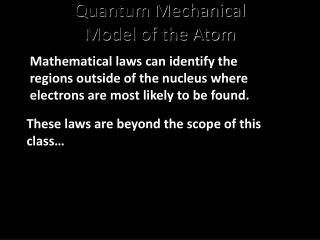DownloadDownload PresentationQuantum Mechanical Model of the Atom

# Quantum Mechanical Model of the Atom

Download Presentation## Quantum Mechanical Model of the Atom

- - - - - - - - - - - - - - - - - - - - - - - - - - - E N D - - - - - - - - - - - - - - - - - - - - - - - - - - -
##### Presentation Transcript

1. Quantum MechanicalModel of the Atom Mathematical laws can identify the regions outside of the nucleus where electrons are most likely to be found. These laws are beyond the scope of this class…

2. Schrodinger Wave Equation Equation for probability of a single electron being found along a single axis (x-axis) Erwin Schrodinger

3. Heisenberg Uncertainty Principle “One cannot simultaneously determine both the position and momentum of an electron.” You can find out where the electron is, but not where it is going. OR… You can find out where the electron is going, but not where it is! Werner Heisenberg

4. QUANTUM NUMBERS We will focus now on polyelectronic atoms – atoms with more than one electron. The shape, size, and energy of each orbital is a function of 3 quantum numbers which describe the location of an electron within an atom or ion n(principal) ---> energy level l (orbital) ---> shape of orbital ml(magnetic) ---> designates a particular suborbital The fourth quantum number is not derived from the wave function s(spin) ---> spin of the electron (clockwise or counterclockwise: ½ or – ½)

5. Principal Quantum Number The principal quantum number, n, denotes the probable distance of an electron from the nucleus. n = energy levels (shells) = 1, 2, 3, 4, …. distance of e- from the nucleus

6. Angular Momentum Quantum Number An orbital is a region within an energy level where there is a probability of finding an electron. The angular momentum quantum number, l, denotes the orbital (subshell or sublevel) in which an electron is located. for a given value of n, l = 0, 1, 2, 3, … n-1 l = 0 s orbital l = 1 p orbital l = 2 d orbital l = 3 f orbital n = 1, l = 0 n = 2, l = 0 or 1 n = 3, l = 0, 1, or 2 Shape of the “volume” of space that the e- occupies

7. s Orbital shape The s orbital (l = 0) has a spherical shape centered around the origin of the three axes in space.

8. p orbital shape Planar node There are three dumbbell-shaped p orbitals (l = 1) in each energy level above n = 1, each assigned to its own axis (x, y and z) in space. Note: there is a planar node through the nucleus, which is a area of zero probability of finding an electron.

9. d orbital shapes Things get a bit more complicated with the five d orbitals (l = 2) that are found in the d sublevels beginning with n = 3. To remember the shapes, think of “doubledumbells” …and a “dumbell with a donut”!

10. Shape of f (l = 3) orbitals

11. Magnetic Quantum Number The magnetic quantum number m, denotes the orientation of an electron’s orbital with respect to the three axes in space. for a given value of l ml = -l, …., 0, …. +l if l = 1 (p orbital), ml = -1, 0, or 1 if l = 2 (d orbital), ml = -2, -1, 0, 1, or 2 orientation of the orbital in space

12. Assigning the Numbers • The three quantum numbers (n, l, and m) are integers. • The principal quantum number (n) cannot be zero. • n must be 1, 2, 3, etc. • The angular momentum quantum number (l ) can be any integer between 0 and n - 1. • For n = 3, l can be either 0, 1, or 2. • The magnetic quantum number (ml) can be any integer between -l and +l. • For l = 2, m can be either -2, -1, 0, +1, +2.

13. Spin Quantum Number The spin quantum number, ms, describes the behavior of an electron in a magnetic field. spin quantum number ms ms = +½ or -½ ms = +½ ms = -½

14. Pauli exclusion principle - no two electrons in an atom can have the same four quantum numbers. Each seat is uniquely identified (E, R12, S8) Each seat can hold only one individual at a time

15. How many electrons can an orbital hold? Shell – electrons with the same value of n Subshell or sublevel – electrons with the same values of nandl Orbital – electrons with the same values of n, l, andml If n, l, and mlare fixed, then ms = ½ or - ½ An orbital can hold 2 electrons

16. How many electrons can be in a sublevel subshell? Remember: A maximum of two electrons can be placed in an orbital. s orbitals p orbitals d orbitals f orbitals Number of orbitals 1 3 5 7 Number of electrons 6 10 14 2